# KSEEB Solutions for Class 8 Maths Chapter 10 Exponents Additional Questions

Students can Download Maths Chapter 10 Exponents Additional Questions and Answers, Notes Pdf, KSEEB Solutions for Class 8 Maths helps you to revise the complete Karnataka State Board Syllabus and score more marks in your examinations.

## Karnataka State Syllabus Class 8 Maths Chapter 10 Exponents Additional Questions

Question 1.
Mark the correct option.
(a) The value of (3m)n, for every pair of integers (m, n) is
A. 3m+n
B. 3mn
C. 3mn
D. 3m + 3n
Solution:
(B) 3mn

(b) If x, y, 2x + $$\frac{\mathbf{y}}{2}$$ are non-zero real numbers then $$\left(2 x+\frac{y}{2}\right)^{-1}\left[(2 x)^{-1}+\left(\frac{y}{2}\right)^{-1}\right]$$ equals.
A. 1
B. x . y-1
C. x-1 . y
D. x-1 . y-1
Solution:
(D) x-1 . y-1

(c) If 2x – 2x-2 = 192 the value of x is
A. 5
B. 6
C. 7
D. 8
Solution:
(D) 8

(d) The number$$\left[6^{\left(6^{6}\right)}\right]^{\frac{1}{6}}$$ is equal to
A. 66
B. 66 – 1
C. 6(65)
D. $$6^{6^{5}}$$
Solution:
(C) 6(65)(e) The number of pairs of positive integers (m, n) such that mn = 25 is
A. 0
B. 1
C. 2
D. More than 2
Solution:
(B) 1

Question 2.
The diameter of the sun is 1.4 x 109 meters and that of the earth is about 1.2678 x 107 meteres. Find the approximate ratio of the diameter of the sun to that of the earth.
Solution:= 1.10427 x 109-7
= 1.10427 x 102
= 1.10427 x 100 = 110 . 427
∴ The ratio is 110 . 427 : 1Question 3.
Find the value of each of the following expressions
(a) (-0.75)3 + (0.3)-3 – $$\left(-\frac{3}{2}\right)^{-3}$$
Solution:(b)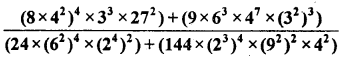Solution: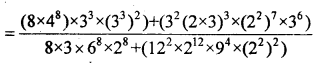(c)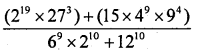Solution: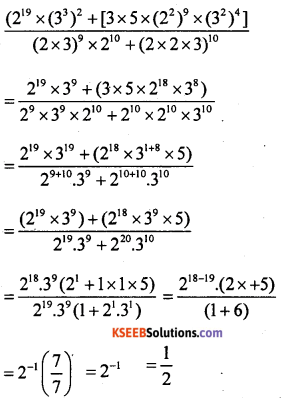Question 4.
How many digits are there in the number 23 x 55 x 205?
Solution:
23 x 55 x 205
= 8 x 625 x 3200000 = 5000 x 32
= 16000000000
∴ The number has 11 digits.Question 5.
If a7 = 3 find the of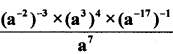Solution: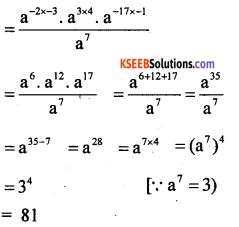Question 6.
If 2m. a2 = 28 where a, m are positive integers find all possible values of a + m.
Solution:
2m . a2 = 28
2m . a2 = 26 . 22
∴m = 6 and a = 2
a + m = 6 + 2 = 8

Question 7.
Suppose 3k x b2 = 64 for some positive integers k and b. Find all possible values of k + b.
Solution:
3k x b2 = 64
= (3 x 2)4
= 34 x 24 = 34 x 22×2 = 34 x (22)2
= 3k x b2 = 34 x 42
∴ k = 4 and b = 4
k + b = 4 + 4 = 8Question 8.
Find the value of $$\frac{(625)^{6.25} \times(25)^{2.60}}{(625)^{7.25} \times(5)^{1.20}}$$
Solution: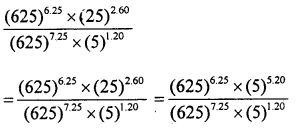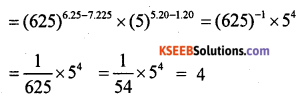Question 9.
A person had some rupees which is a power of 5. He gave a part of it to his friend which is also a power of 5. He was left with Rs. 500. How much did money he have?
Solution:
The powers of 5 are 51 = 5, 52 = 25, 53
= 125, 54 = 625,
55 = 3125 etc.
We find that 625 – 125 = 500
∴ The money with him was Rs. 625.error: Content is protected !!